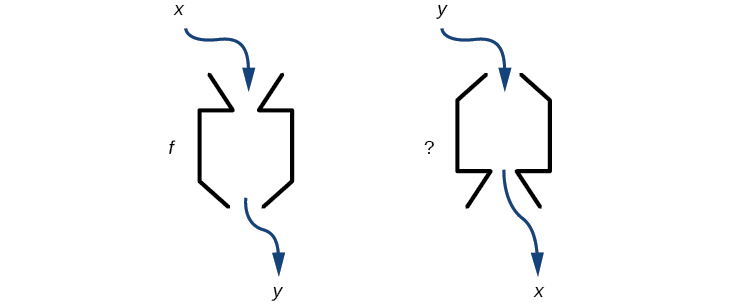# 3.7 Inverse functions

 Page 1 / 9
In this section, you will:
• Verify inverse functions.
• Determine the domain and range of an inverse function, and restrict the domain of a function to make it one-to-one.
• Find or evaluate the inverse of a function.
• Use the graph of a one-to-one function to graph its inverse function on the same axes.

A reversible heat pump is a climate-control system that is an air conditioner and a heater in a single device. Operated in one direction, it pumps heat out of a house to provide cooling. Operating in reverse, it pumps heat into the building from the outside, even in cool weather, to provide heating. As a heater, a heat pump is several times more efficient than conventional electrical resistance heating.

If some physical machines can run in two directions, we might ask whether some of the function “machines” we have been studying can also run backwards. [link] provides a visual representation of this question. In this section, we will consider the reverse nature of functions.Can a function “machine” operate in reverse?

## Verifying that two functions are inverse functions

Suppose a fashion designer traveling to Milan for a fashion show wants to know what the temperature will be. He is not familiar with the Celsius scale. To get an idea of how temperature measurements are related, he asks his assistant, Betty, to convert 75 degrees Fahrenheit to degrees Celsius. She finds the formula

$C=\frac{5}{9}\left(F-32\right)$

and substitutes 75 for $\text{\hspace{0.17em}}F\text{\hspace{0.17em}}$ to calculate

$\frac{5}{9}\left(75-32\right)\approx 24\text{°C}$

Knowing that a comfortable 75 degrees Fahrenheit is about 24 degrees Celsius, he sends his assistant the week’s weather forecast from [link] for Milan, and asks her to convert all of the temperatures to degrees Fahrenheit.

At first, Betty considers using the formula she has already found to complete the conversions. After all, she knows her algebra, and can easily solve the equation for $\text{\hspace{0.17em}}F\text{\hspace{0.17em}}$ after substituting a value for $\text{\hspace{0.17em}}C.\text{\hspace{0.17em}}$ For example, to convert 26 degrees Celsius, she could write

$\begin{array}{ccc}\hfill 26& =& \frac{5}{9}\left(F-32\right)\hfill \\ \hfill 26\cdot \frac{9}{5}& =& F-32\hfill \\ \hfill F& =& 26\cdot \frac{9}{5}+32\approx 79\hfill \end{array}$

After considering this option for a moment, however, she realizes that solving the equation for each of the temperatures will be awfully tedious. She realizes that since evaluation is easier than solving, it would be much more convenient to have a different formula, one that takes the Celsius temperature and outputs the Fahrenheit temperature.

The formula for which Betty is searching corresponds to the idea of an inverse function , which is a function for which the input of the original function becomes the output of the inverse function and the output of the original function becomes the input of the inverse function.

Given a function $\text{\hspace{0.17em}}f\left(x\right),\text{\hspace{0.17em}}$ we represent its inverse as $\text{\hspace{0.17em}}{f}^{-1}\left(x\right),\text{\hspace{0.17em}}$ read as $\text{\hspace{0.17em}}“f\text{\hspace{0.17em}}$ inverse of $\text{\hspace{0.17em}}x.\text{”}\text{\hspace{0.17em}}$ The raised $\text{\hspace{0.17em}}-1\text{\hspace{0.17em}}$ is part of the notation. It is not an exponent; it does not imply a power of $\text{\hspace{0.17em}}-1\text{\hspace{0.17em}}$ . In other words, $\text{\hspace{0.17em}}{f}^{-1}\left(x\right)\text{\hspace{0.17em}}$ does not mean $\text{\hspace{0.17em}}\frac{1}{f\left(x\right)}\text{\hspace{0.17em}}$ because $\text{\hspace{0.17em}}\frac{1}{f\left(x\right)}\text{\hspace{0.17em}}$ is the reciprocal of $\text{\hspace{0.17em}}f\text{\hspace{0.17em}}$ and not the inverse.

The “exponent-like” notation comes from an analogy between function composition and multiplication: just as $\text{\hspace{0.17em}}{a}^{-1}a=1\text{\hspace{0.17em}}$ (1 is the identity element for multiplication) for any nonzero number $\text{\hspace{0.17em}}a,\text{\hspace{0.17em}}$ so $\text{\hspace{0.17em}}{f}^{-1}\circ f\text{\hspace{0.17em}}$ equals the identity function, that is,

#### Questions & Answers

A laser rangefinder is locked on a comet approaching Earth. The distance g(x), in kilometers, of the comet after x days, for x in the interval 0 to 30 days, is given by g(x)=250,000csc(π30x). Graph g(x) on the interval [0, 35]. Evaluate g(5)  and interpret the information. What is the minimum distance between the comet and Earth? When does this occur? To which constant in the equation does this correspond? Find and discuss the meaning of any vertical asymptotes.
Kaitlyn Reply
The sequence is {1,-1,1-1.....} has
amit Reply
circular region of radious
Kainat Reply
how can we solve this problem
Joel Reply
Sin(A+B) = sinBcosA+cosBsinA
Eseka Reply
Prove it
Eseka
Please prove it
Eseka
hi
Joel
June needs 45 gallons of punch. 2 different coolers. Bigger cooler is 5 times as large as smaller cooler. How many gallons in each cooler?
Arleathia Reply
7.5 and 37.5
Nando
find the sum of 28th term of the AP 3+10+17+---------
Prince Reply
I think you should say "28 terms" instead of "28th term"
Vedant
the 28th term is 175
Nando
192
Kenneth
if sequence sn is a such that sn>0 for all n and lim sn=0than prove that lim (s1 s2............ sn) ke hole power n =n
SANDESH Reply
write down the polynomial function with root 1/3,2,-3 with solution
Gift Reply
if A and B are subspaces of V prove that (A+B)/B=A/(A-B)
Pream Reply
write down the value of each of the following in surd form a)cos(-65°) b)sin(-180°)c)tan(225°)d)tan(135°)
Oroke Reply
Prove that (sinA/1-cosA - 1-cosA/sinA) (cosA/1-sinA - 1-sinA/cosA) = 4
kiruba Reply
what is the answer to dividing negative index
Morosi Reply
In a triangle ABC prove that. (b+c)cosA+(c+a)cosB+(a+b)cisC=a+b+c.
Shivam Reply
give me the waec 2019 questions
Aaron Reply

### Read also:

#### Get the best Algebra and trigonometry course in your pocket!

Source:  OpenStax, Algebra and trigonometry. OpenStax CNX. Nov 14, 2016 Download for free at https://legacy.cnx.org/content/col11758/1.6
Google Play and the Google Play logo are trademarks of Google Inc.

Notification Switch

Would you like to follow the 'Algebra and trigonometry' conversation and receive update notifications?ByByBy